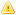HelpWLanguageWLanguage functionsStandard functionsNumeric values management functionsBinary values management functionsBinaryORPresentationExampleBitwise calculationBinary operatorSee alsoBinary values management functionsBinaryANDBinaryNOTBinaryORBinaryXORThis content has been translated automatically.  Click here  to view the French version.
• Bitwise calculation
• Binary operator
WINDEVWEBDEVWINDEV MobileOthersBinaryOR (Function)
In french: OUBinaire
Returns the result of the (bitwise) logical OR between two values.
Example
// Récupération du OU logique
ResOUBinaire = BinaryOR(6, 6)  // Renvoie 6
ResOUBinaire = BinaryOR(6, 2)  // Renvoie 6
Syntax
<Result> = BinaryOR(<Value 1> , <Value 2>)
<Result>: Integer
Result of logical OR.
<Value 1>: Integer
First value to test.
<Value 2>: Integer
Second value to test.
Remarks

Bitwise calculation

BinaryOR performs the bitwise calculation between <Value 1> and <Value 2> based on the following table:
 0 1 0 0 1 1 1 1

Binary operator

• The function BinaryOR is equivalent to the binary operator | for 4-byte integers. For more details, see Binary operators.
• The result of the BinaryOR function is exact on 4-byte integers. Its use with 8-byte integers causes a capacity overflow.
• The binary operator | allows you to perform operations on 8-byte integers.
Component: wd280mat.dll
Minimum version required
• Version 9